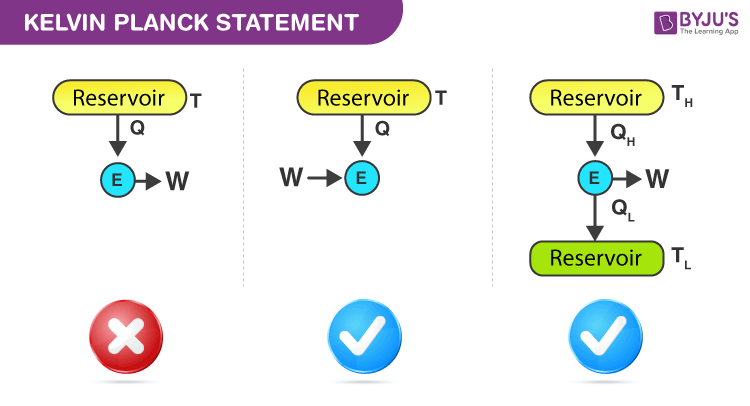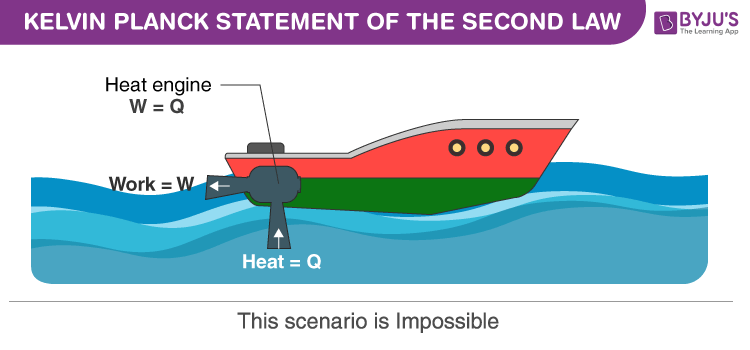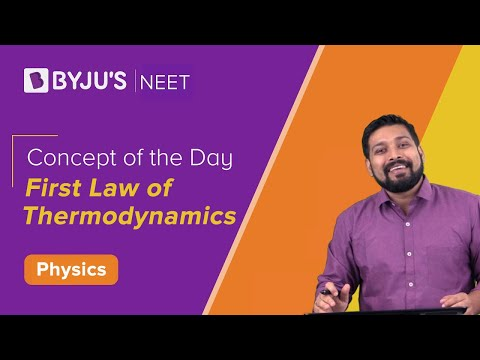Checkout JEE MAINS 2022 Question Paper Analysis : Checkout JEE MAINS 2022 Question Paper Analysis :

# Kelvin-Planck Statement

## What is the Kelvin Planck Statement?

This is a special case of the second law of thermodynamics. It states that “It is impossible to construct a device which operates on a cycle and produces no other effect than the transfer of heat from a single body to produce work.” This means that it is impossible to construct an engine whose sole purpose is to convert the heat from a high-temperature source/reservoir into an equal amount of work.We know that heat (Q) and Work(W) are the two forms of energy. Both follow the S.I unit Joules, and both are interconvertible. This means work can be converted into work and vice-versa. Here, work can be fully converted into heat, but heat cannot be fully converted into work. Hence, work is called high-grade energy, and heat is called low-grade energy.

The Kelvin-Planck statement is two different statements given by Lord Kelvin and Planck. They are known as Kelvin’s statement and Planck’s statement.

Kelvin’s statement stated that it is impossible to derive mechanical effect from any matter by cooling it below the highest cooling temperature of the surrounding objects.

Planck’s statement states that the total sum of entropies for the reversible system remains constant.

Clubbing both these statements, the Kelvin-Planck statement was derived.

The kelvin-Planck statement, also known as the heat engine statement from the second law of thermodynamics, states that it is impossible to design a device that works on a cycle and produces no other effect other than heat transfer from a single body for the production of work.## Kelvin-Planck Statement Example

A hypothetical device called the perpetual motion machine of the second kind (PMMSK) was introduced by Wilhelm Ostwald, which would perform work exclusively by absorbing energy as heat from a body.

### Working of Heat Engine:

For the operation of the heat engine, the working fluid has to continuously exchange heat between the heat sink/reservoir with low temperature

$$\begin{array}{l}Q_{L}\end{array}$$
and the heat source/reservoir with high temperature
$$\begin{array}{l}Q_{H}\end{array}$$
. The prime parameter involved here is efficiency.

The efficiency of a heat engine is “The amount of useful  work obtained(output) for a given amount of input

In general, efficiency tells the heat transfer process. It is the ratio of “how much you get out” to “how much you put in”

Efficiency

$$\begin{array}{l}\eta =\frac{-W}{-Q_{H}}\end{array}$$

$$\begin{array}{l}=\frac{Q_{L}-Q_{H}}{Q_{L}}\end{array}$$

$$\begin{array}{l}=1-\frac{Q_{L}}{Q_{H}}\end{array}$$

In the absence of a heat sink, that is:

$$\begin{array}{l}Q_{L}=0\end{array}$$
. Then efficiency

$$\begin{array}{l}\eta =1-\frac{0}{Q_{H}}=1\end{array}$$

This implies that efficiency is 100%. Which is not true according to the second law of thermodynamics. Thus, no heat engine is 100% efficient.

 $$\begin{array}{l}\eta<1\end{array}$$

## Real-Life Scenario:

The real-life applications of thermodynamics are plenty. To move the boat, heat energy is converted into work energy. The water body comprises an enormous amount of heat. So ideally, we can extract heat from the water body and convert that heat completely into propulsion to work. Unfortunately, in a practical scenario, it does not work. A well-equipped engine with fuel is essential to move the boat forward. This works as an external heat supply for the functioning of propulsion. Thus, we realize that it is impossible to build an engine that converts heat from one reservoir completely into work. This aids the Kelvin Planck statement.

Related Physics Articles:

## Watch the video and learn the first law of thermodynamics.Get registered to BYJU’S-The learning app to receive the latest set of interactive physics videos. To know more about laws of thermodynamics and its applications, stay tuned with BYJU’S.

Test your knowledge on Kelvin Planck Statement